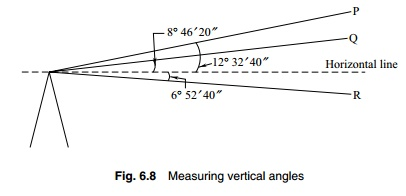Home | | Surveying I | | Surveying | Measuring Vertical Angles in Theodolite

# Measuring Vertical Angles in TheodoliteA vertical angle is made by an inclined line of sight with the horizontal. The line of sight may be inclined upwards or downwards from the horizontal.

Measuring Vertical Angles in Theodolite

A vertical angle is made by an inclined line of sight with the horizontal. The line of sight may be inclined upwards or downwards from the horizontal. Thus one may have an angle of elevation or depression. See Fig. 6.8. For measuring vertical angles, the theodolite is levelled with respect to the altitude bubble.1.  Set up the theodolite at the station from where the vertical angle is to be mea-sured. Level the instrument with reference to the plate bubble.

2 . further level the instrument with respect to the altitude level ixed on the index rm. This bubble is generally more sensitive. The procedure for levelling is the same. Bring the altitude level parallel to two foot screws and level till the bubble traverses. Swing through 90 o to centre the bubble again with the third foot screw. Repeat till the bubble traverses.

3.  Swing the telescope to approximately direct the line of sight towards the signal at P. Loosen the vertical circle clamp screw and incline the line of sight to bisect P. Clamp the vertical circle and bisect the signal exactly with the horizontal cross hair.

4.  Read the verniers C and D. The average of these readings gives the value of the angle.

This procedure assumes that the instrument is properly adjusted. If there is an index error, the instrument does not read zero when the bubble is in the centre and the line of sight is horizontal, the adjustment is done by the clip screw. There may be a small index error, which can be accounted for in the value of angle. The readings can be recorded as shown in Table 6.3.

Measuring Vertical Angle Between Two Points

The two points may be above the horizontal or below the horizontal or one may be above and the other below. In all cases, the vertical angles between the instrument and the points are measured. If the points lie on the same side of the horizontal, the vertical angle between the points is the difference between the measured angles. If they lie on either side of the horizontal through the instrument, the vertical angle between the points is the sum of the angles measured.

Table 6.7  Recording of observations Face leftInterconversion of Angles

The theodolite measures the whole circle bearings of lines. These can be converted to reduced bearings by the methods discussed in Chapter 3. Also, one can calculate included angles from bearings and vice versa. Included angles can also be calculated from deflection angles and vice versa.

The following relationships of the angles of a closed traverse are known from geometry:

(a)  sum of the interior angles = (2n - 4) right angles

(b) sum of exterior angles = (2n + 4) right angles

(c)  sum of the deflection angles = 4 right angles

It is desirable to draw a rough sketch of the traverse before attempting to solve problems. The following examples illustrate these principles.

Study Material, Lecturing Notes, Assignment, Reference, Wiki description explanation, brief detail
Civil Surveying - Levelling and Applications : Measuring Vertical Angles in Theodolite |

Related Topics